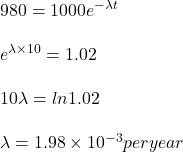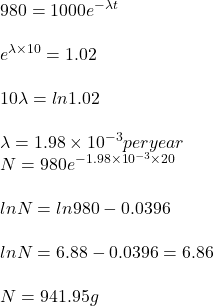## A radioactive material is known to decay at a yearly rate proportional to the amount at each moment. There were 1000 grams of the material 1

Question

A radioactive material is known to decay at a yearly rate proportional to the amount at each moment. There were 1000 grams of the material 10 years ago. There are 980 prams right now. What will be the amount of the material right after 20 years?
a. 10 ln 2/ln(1000/980)
b. 10^6/980
c. 980^3/10^6
d. 980^2/10^3

in progress 0
2 months 2021-07-24T15:14:27+00:00 1 Answers 4 views 0

Amount left is 941.95 g.

Step-by-step explanation:

initial amount = 1000 g

time = 10 years

amount left =  980 grams

Nowtime t = 20 years

Let the amount is N.